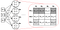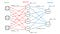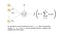# The Mathematics of Neural Networks

So my last article was a very basic description of the MLP. In this article, I’ll be dealing with all the mathematics involved in the MLP. For those who haven’t read the previous article, you can read it here. Without any waste of time, let’s dive in.

# The Basics

The first thing you have to know about the Neural Network math is that it’s very simple and anybody can solve it with pen, paper, and calculator (not that you’d want to). However, you could have more than hundreds of thousands of neurons, so it could take forever to solve. Secondly, a bulk of the calculations involves matrices. If you’re not comfortable with matrices, you can find a great write-up here, it’s quite explanatory.

## 1. Weights

As highlighted in the previous article, a weight is a connection between neurons that carries a value. The higher the value, the larger the weight, and the more importance we attach to neuron on the input side of the weight. Also, in math and programming, we view the weights in a matrix format. Let’s illustrate with an image.Image taken from here

As you can see in the image, the input layer has 3 neurons and the very next layer (a hidden layer) has 4. We can create a matrix of 3 rows and 4 columns and insert the values of each weight in the matrix as done above. This matrix would be called W1. In the case where we have more layers, we would have more weight matrices, W2, W3, etc.
In general, if a layer L has N neurons and and the next layer L+1 has M neurons, the weight matrix is an N-by-M matrix (N rows and M columns).

Again, look closely at the image, you’d discover that the largest number in the matrix is W22 which carries a value of 9. Our W22 connects IN2 at the input layer to N2 at the hidden layer. This means that at this state” or currently, our N2 thinks that the input IN2 is the most important of all 3 inputs it has received in making its own tiny decision.

## 2. Bias

The bias is also a weight. Imagine you’re thinking about a situation (trying to make a decision). You have to think about all possible (or observable) factors. But what about parameters you haven’t come across? What about factors you haven’t considered? In a Neural Net, we try to cater for these unforeseen or non-observable factors. This is the bias. Every neuron that is not on the input layer has a bias attached to it, and the bias, just like the weight, carries a value. The image below is a good illustration.Image taken from here

Looking carefully at the layer in the hidden and output layers (with 4 and 2 neurons respectively), you’ll find that each neuron has a tiny red/blue arrow pointing at it. You’ll also discover that these tiny arrows have no source neuron.

Just like weights can be viewed as a matrix, biases can also be seen as matrices with 1 column (a vector if you please). As an example, the bias for the hidden layer above would be expressed as [[0.13], [0.14], [0.15], [0.16]].

## 3. Activation

For this section, let’s focus on a single neuron. After aggregating all the input into it, let’s call this aggregation z (don’t worry about the aggregation, I’ll explain later. For now, just represent everything coming into the neuron as z), a neuron is supposed to make a tiny decision on that output and return another output. This process (or function) is called an activation. We represent it as f(z), where z is the aggregation of all the input. There are 2 broad categories of activation, linear and non-linear. If f(z)=z, we say the f(z) is a linear activation (i.e nothing happens). The rest are non-linear and are described below.Image taken from here

There are several ways our neuron can make a decision, several choices of what f(z) could be. A few popular ones are highlighted here:

• Rectified Linear Units (ReLU) — With ReLU, we ensure our output doesn’t go below zero (or negative). Therefore if z is greater than zero, our output remains z, else if z is negative, our output is zero. The formula is f(z) = max(0, z). Long story short, we select the maximum between 0 and z. Here’s a lovely read on ReLU.
• Tanh — Here, our f(z) = tanh(z). It’s that simple. We find the hyperbolic tangent of z and return it. Don’t worry, your scientific calculator can do this.
• Sigmoid activation — This time, we use the formula:
f(x) = 1 / (1 + e^(-1*z)). Follow the following steps:
1. Negate z by multiplying by -1.
2. Find the exponent of the output in 1. Again, your calculator can do this.
3. Add 1 to the output in 2.
4. Divide 1 by the output in 3.
That’s all.

Note that there are more non-linear activation functions, these just happen to be the most widely used. Also, the choice of the function is heavily dependent on the problem you’re trying to solve or what your NN is attempting to learn.

# The Mathematics

Now that you know the basics, it’s time to do the math. Remember this?

Yea, you saw that in the image about activation functions above. Here’s the explanation on aggregation I promised:

See everything in the parentheses? Call that your z. Then;

• b = bias
• x = input to neuron
• w = weights
• n = the number of inputs from the incoming layer
• i = a counter from 0 to n

Before we go further, note that ‘initially’, the only neurons that have values attached to them are the input neurons on the input layer (they are the values observed from the data we’re using to train the network). So, how does this work?

1. Multiply every incoming neuron by its corresponding weight.
2. Add the values up.
3. Add the bias term for the neuron in question.

That’s all for evaluating z for our neuron. Simple right?

But imagine you have to do this for every neuron (of which you may have thousands) in every layer (of which you might have hundreds), it would take forever to solve. So here’s the trick we use:

Remember the matrices (and vectors) we talked about? Here’s when we get to use them. Follow these steps:

1. Create a weight matrix from input layer to the output layer as described earlier; e.g. N-by-M matrix.
2. Create an M-by-1 matrix from the biases.
3. View your input layer as an N-by-1 matrix (or vector of size N, just like the bias).
4. Transpose the weight matrix, now we have an M-by-N matrix.
5. Find the dot product of the transposed weights and the input. According to the dot-product rules, if you find the dot product of an M-by-N matrix and an N-by-1 matrix, you get an M-by-1 matrix.
6. Add the output of step 5 to the bias matrix (they will definitely have the same size if you did everything right).
7. Finally, you have the values of the neurons, it should be an M-by-1 matrix (vector of size M)

After all that, run the activation function of your choice on each value in the vector.

Do this for every weight matrix you have, finding the values of the neurons/units as you go forward. Continue until you get to the end of the network (the output layer).

That’s it. But not the end. There’s the the part where we calculate how far we are from the original output and where we attempt to correct our errors. I will described these in upcoming articles.

WARNING: This methodology works for fully-connected networks only. The weight matrices for other types of networks are different.

Now, you can build a Neural Network and calculate it’s output based on some given input. As you can see, it’s very very easy. Give yourself a pat on the back and get an ice-cream, not everyone can do this.

Thanks for reading this, watch out for upcoming articles because you’re not quite done yet.

Written by

## Coinmonks

#### Coinmonks is a technology-focused publication embracing decentralize technologies. We are Non-profit and education is our core value. Learn, Build and thrive. Our other project— https://coincodecap.com

Welcome to a place where words matter. On Medium, smart voices and original ideas take center stage - with no ads in sight. Watch
Follow all the topics you care about, and we’ll deliver the best stories for you to your homepage and inbox. Explore
Get unlimited access to the best stories on Medium — and support writers while you’re at it. Just \$5/month. Upgrade